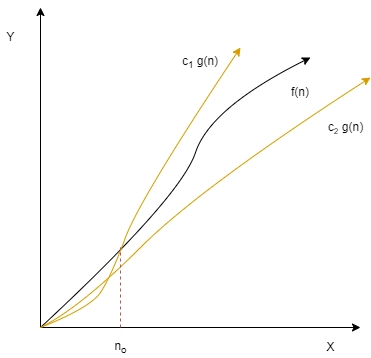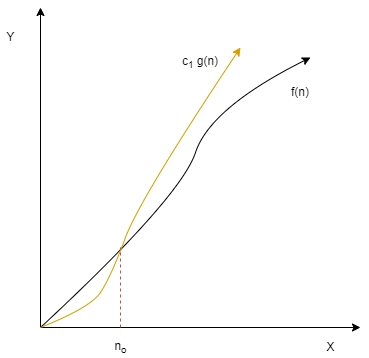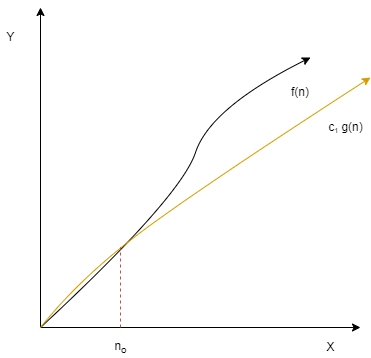New update is available. Click here to update.
Last Updated: Sep 27, 2023

# Asymptotic Notations

## Introduction

Asymptotic notations are mathematical tools that help us study the asymptotic behaviour of functions. They can help us analyse algorithms independently of the machine architecture, OS being used or the compiler configuration. Understanding the space and time complexity of programs using these tools doesn't require us to run the codes on machines that can give varying observations depending on various factors and help us identify algorithms with better performance (in terms of space or time) without any ambiguity. There are three asymptotic notations that are popularly used:

1. Ө Notation or Big Theta (Ө) Notation
2. Big-O Notation
3. Ω Notation or Big Omega notation

### Ө(Big Theta) Notation

Let there be a function f(n), n >= 0.

Now if there’s a function g(n), n >= 0, let the c1g(n) >= f(n) >= c2g(n)  for ∀ n >= no and c1, c2 > 0.Then, f(n) = Ө(g(n)), here g(n) is the asymptotically tight bound on the function f(n).

The above definition helps you understand the theory behind this notation but finding a meaningful function g(n) is more important to analyse f(n).

If f(n) is a polynomial (axnx + ax-1x-1 + .. + a1n1 + a0), then g(n) can be nx. You can always find constants c1 and c2 such that the previously defined conditions are satisfied because for a large enough n,  nx > c * nx-k ∀ (x >= k >= 1, c3 > 0).

For example if f(n) = n5 + 3n4 + 7n2 + 1, then g(n) = n5 is a valid choice.

### Big-O Notation

Let there be a function f(n), n >= 0.

Now if there’s a function g(n), n >= 0, let the c g(n) >= f(n) for ∀ n >= no and c > 0.Then, f(n) = O(g(n)), here g(n) is the upper bound on the function f(n).

The above has helps us again in understanding the theory behind this notation, unlike Ө notation, finding the upper bound on a function is often easier and helps us find the worst case complexity of our algorithm. Here if f(n) = Ө(g(n)), then f(n) = O(g(n)). Therefore, if f(n) is a polynomial (axnx + ax-1x-1 + .. + a1n1 + a0) then we can select g(n) = nx + k, k > 0.

For example if f(n) = n5 + 3n4 + 7n2 + 1, then g(n) = n7 is a valid choice.

### Ω(Big Omega) Notation

Let there be a function f(n), n >= 0.

Now if there’s a function g(n), n >= 0, let the f(n) >= c g(n) for ∀ n >= no and c > 0.Then, f(n) = Ω(g(n)), here g(n) is the lower bound on the function f(n).

Unlike θ and Big-O notation, finding the lower bound on a function is not that useful (we usually need the average and the worst-case complexity), and the Ω notation is one of the least used notations compared to the earlier two.

Here again if f(n) = θ(g(n)), then f(n) = Ω(g(n)). If f(n) is a polynomial (axnx + ax-1x-1 + .. + a1n1 + a0) then we can select g(n) = nx - k + 1 , x + 1 >= k > 0.

For example if f(n) = n5 + 3n4 + 7n2 + 1, then g(n) = n4 is a valid choice.

Also see, Longest Common Substring and Data Structure

Must Read Lower Bound in C++

## Properties of Asymptotic Notations

Assume that the below properties hold for all notations big-O, θ and Ω notations.

Basic Properties:

1. If f(n) = O(g(n))
and let, h(n) = a * g(n) for a > 0.
then, f(n) = O(h(n))
and
2. If f(n) = O(g(n))
and let, h(n) = a * f(n) for a > 0.
then, h(n) = O(g(n))

Reflexive, Symmetric, Transpose Symmetric and Transitive:

1. It’s reflexive, because f(n) = O(f(n)), this is true because f(n) is the upper as well as the lower bound of itself.
2. Symmetric property is only valid for θ notation and here if f(n) = θ(g(n)), then g(n) = θ(f(n)).
3. Transpose Symmetric property is only valid for big-O and θ notation and here if f(n) = O(g(n)), then g(n) = Ω(f(n)).
4. It’s transitive, If f(n) = O(g(n)) and g(n) = O(h(n)), then f(n) = O(h(n)).

Commonly used properties:

1. If f(n) = O(g(n)) and f(n) = Ω(g(n)), then f(n) = θ(g(n)).
2. Here if, f(n) = O(h(n)) and g(n) = O(k(n)).

Then, f(n) + g(n) = O(max(h(n), k(n)))

And similarly,

f(n) * g(n) = O(h(n) * k(n))

## FAQs

1. What is Time complexity?
It measures the time consumed by an algorithm to produce an output given a particular input of known size.

2. What is Space Complexity?
It is the measure of memory consumed by an algorithm to operate on the input of a given size. The space complexity is the sum of the auxiliary and the input memory consumed.

3. What does an asymptote mean?
Asymptote refers to lines that are tangent to a particular curve at infinity, i.e., Limn->∞(f(n)/g(n)) = 1 for these two functions in this case. Therefore, these two functions can also be said to be asymptotically equivalent.

4. What is Auxiliary memory?
Auxiliary memory refers to the cheap, slow and large memory units such as HDDs in our computer. Most of the information related to a program is stored for long term storage when not being used.

5. Why is the best case time complexity less useful than the worst case or average case?
Finding the worst-case time complexity ensures that our algorithm runs within the time window. This is relevant when we ensure that no operation exceeds some hard time limit. The average case time can be helpful when we have to decide the better algorithm for a task in practice (like quicksort for sorting). On the other hand, the best case doesn't provide enough information to make similar decisions.

## Key Takeaways

This article covers the essentials for understanding asymptotic notations and their primary uses as mathematical tools to analyse algorithms' space and time complexity without being dependent on the OS, hardware, etc. To understand the blog better, refer to the content here about time complexity analysis, and refer here to learn more about space and time complexity.

Read More - Time Complexity of Sorting Algorithms

Learn more about the C++ STL library from here if you have trouble understanding some part of the code. Visit the link here for carefully crafted courses on campus placement and interview preparation on coding ninjas.

Happy learning!